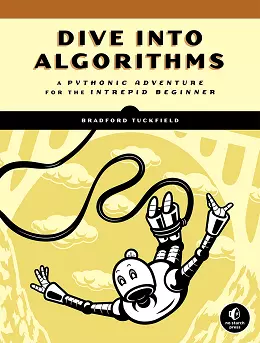# Dive Into Algorithms### eBook Details:

• Paperback: 248 pages
• Publisher: WOW! eBook (January 5, 2021)
• Language: English
• ISBN-10: 1718500688
• ISBN-13: 978-1718500686

### eBook Description:

Dive Into Algorithms: A Pythonic Adventure for the Intrepid Beginner

Dive Into Algorithms is a wide-ranging, Pythonic tour of many of the world’s most interesting algorithms. With little more than a bit of computer programming experience and basic high-school math, you’ll explore standard computer science algorithms for searching, sorting, and optimization; human-based algorithms that help us determine how to catch a baseball or eat the right amount at a buffet; and advanced algorithms like ones used in machine learning and artificial intelligence. You’ll even explore how ancient Egyptians and Russian peasants used algorithms to multiply numbers, how the ancient Greeks used them to find greatest common divisors, and how Japanese scholars in the age of samurai designed algorithms capable of generating magic squares.

You’ll explore algorithms that are useful in pure mathematics and learn how mathematical ideas can improve algorithms. You’ll learn about an algorithm for generating continued fractions, one for quick calculations of square roots, and another for generating seemingly random sets of numbers.

You’ll also learn how to:

• Use algorithms to debug code, maximize revenue, schedule tasks, and create decision trees
• Measure the efficiency and speed of algorithms
• Generate Voronoi diagrams for use in various geometric applications
• Use algorithms to build a simple chatbot, win at board games, or solve sudoku puzzles
• Write code for gradient ascent and descent algorithms that can find the maxima and minima of functions
• Use simulated annealing to perform global optimization
• Build a decision tree to predict happiness based on a person’s characteristics

Once you’ve finished this Dive Into Algorithms: A Pythonic Adventure for the Intrepid Beginner book you’ll understand how to code and implement important algorithms as well as how to measure and optimize their performance, all while learning the nitty-gritty details of today’s most powerful algorithms.